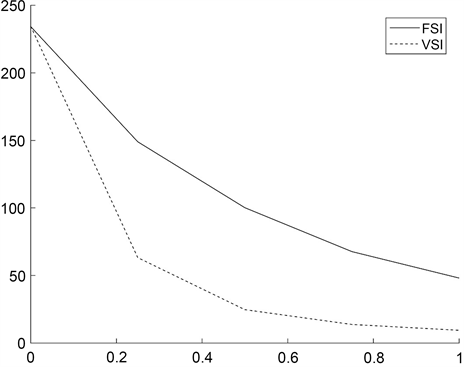#### 期刊菜单

Comparison of FSI CUSUM and VSI CUSUM Control Charts under Chi-Square Distribution
DOI: 10.12677/SA.2022.112045, PDF, HTML, XML, 下载: 48  浏览: 75

Abstract: In this paper, the average time to signal is chosen to measure the monitoring efficiency of VSI CUSUM control chart, and the parameters of FSI CUSUM control chart and VSI CUSUM control chart are adjusted and optimized under the standard normal distribution, and the monitoring efficiency of FSI CUSUM control chart and VSI CUSUM control chart under the chi-square distribution are compared.

1. 引言

2. CUSUM控制图的参数确定

2.1. CUSUM控制图的设计

${Z}_{j}=\mathrm{max}\left\{0,{Z}_{j-1}+{X}_{j}+k\right\}$

$F=f\cdot {\sigma }_{0}$

$H=h\cdot {\sigma }_{0}$

${F}_{1}={f}_{1}\cdot {\sigma }_{0}$

${H}_{1}={h}_{1}\cdot {\sigma }_{0}$

VSI CUSUM控制图中还需要设定上下警戒限，斜率 ${F}_{2}$ 可以写为:

${F}_{2}={f}_{2}\cdot {\sigma }_{0}$

${H}_{2}={h}_{2}\cdot {\sigma }_{0}$

${I}_{1}=\left(0,{H}_{2}\right)$ 为中心域；

${I}_{2}=\left({H}_{2},{H}_{1}\right)$ 为警戒域。

$0<|{Z}_{t}|\le {H}_{2}$，即观测点落在中心域，说明产品质量良好，此时可以采取宽松的政策，也就是延长下一次抽样的时间，增大样本抽样间隔，即下一个抽样间隔为 ${T}_{1}$

${H}_{2}<|{Z}_{t}|\le {H}_{1}$，即观测点落在警戒域，说明产品质量情况不稳定，有落在控制限之外的可能性，此时需要引起警惕，为了尽快发现偏差出现的原因是否具有随机性，需要缩短下一次抽样的时间，减小样本抽样间隔，即下一个抽样间隔为 ${T}_{2}$

$|{Z}_{t}|>{H}_{1}$，即观测点落在控制限之外，说明产品生产过程中出现了严重问题，此时报警。

2.2. 控制图比较标准

$\text{ATS}=E\left(T\right)\cdot \text{ARL}$

$E\left(T\right)={T}_{1}\cdot p+{T}_{2}\cdot \left(1-p\right)$

2.3. CUSUM控制图参数的确定Table 1. ARL values corresponding to different h valuesTable 2. ARL values corresponding to different h values

${h}_{1}=6.75$${h}_{2}=6.65$ 时，利用随机模拟可以计算观测点落入警戒域的概率p为0.0032，在ATS值为370和 $E\left(T\right)$ 值接近1的条件下，可得 ${T}_{1}=0.99$${T}_{2}=0.4685$，此时 $E\left(T\right)=0.9884$ 与固定样本抽样区间控制图的抽样间隔1大致相等，满足生产成本要求。

3. 卡方分布下两种控制图的比较

3.1. CUSUM控制图

$E\left({X}_{t}\right)={\mu }_{0}=v$

$Var\left({X}_{t}\right)={\sigma }_{0}^{2}=2v$

CUSUM控制图的统计量可以写为：

${Z}_{t}=\mathrm{max}\left\{0,{Z}_{t-1}+{X}_{t}+k\right\}$

${F}_{1}={f}_{1}\cdot {\sigma }_{0}={f}_{1}\cdot \sqrt{2v}$

${H}_{1}={h}_{1}\cdot {\sigma }_{0}={h}_{1}\cdot \sqrt{2v}$

${F}_{2}={f}_{2}\cdot {\sigma }_{0}={f}_{2}\cdot \sqrt{2v}$

${H}_{2}={h}_{2}\cdot {\sigma }_{0}={h}_{2}\cdot \sqrt{2v}$

3.2. 两种控制图的的比较Table 3. ARL and ATS values at different offsetsFigure 1. ARL and ATS values at different offsetsTable 4. ARL and ATS values at different offsetsFigure 2. ARL and ATS values at different offsets

4. 结论

  许志良, 朱永忠. 两种多元变异系数的自适应CUSUM控制图[J]. 信息技术, 2021(7): 1-8, 14.  王芝珺, 雷骏峰, 吴纯杰. 基于对数似然比的多元加权Poisson CUSUM控制图研究及应用[J]. 系统科学与数学, 2021, 41(3): 837-853.  毛樑. 基于正态变换的CUSUM控制图应用研究[D]: [硕士学位论文]. 上海: 华东师范大学, 2015.  朱永忠, 王室壹. 监控过程方差无参数多变量CUSUM控制图设计[J]. 统计与决策, 2021, 37(5): 49-53.  张华初, 楚鹏飞, 谢观霞. 统计分布和中心极限定理的随机模拟[J]. 统计与决策, 2021, 37(4): 69-72.